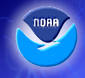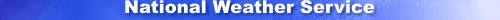Home News Organization Search

 NCEP Home > EMC Home > BUFRLIB > BUFRLIB Table of Contents > Decoding wind profiler BUFR messagesPrinter Friendly Version

# Example Program: Decoding wind profiler BUFR messages

In this example, we will be decoding (i.e. reading) BUFR messages that were encoded according to the "WIND PROFILER" format listed within our sample DX BUFR tables file. In effect, this means that we will be reading data subsets that are described by the Table A mnemonic "NC002007". In doing so, we will pass each BUFR message directly from the application program to the BUFRLIB software via an array in memory, since this will allow us to demonstrate the use of subroutine READERME in lieu of subroutine READMG:

```        PARAMETER       ( MXMN = 7 )
PARAMETER       ( MXLV = 86 )

REAL*8          r8arr ( MXMN, MXLV )

PARAMETER       ( MXBF = 16000 )

CHARACTER	cbfmsg*(MXBF),
+			csubset*8

INTEGER		ibfmsg ( MXBF / 4 )

EQUIVALENCE	( cbfmsg (1), ibfmsg (1) )
C*-----------------------------------------------------------------------

C*	Open the BUFR messages file.

OPEN  ( UNIT = 11, FILE = 'dummy' )

C*	Open the BUFR tables file.

OPEN  ( UNIT = 12, FILE = 'bufrtab.example' )

C*	Associate the tables file with the messages file, and identify
C*	the latter to the BUFRLIB software.

CALL OPENBF  ( 11, 'IN', 12 )

C*	Specify that we would like IDATE values returned using 10 digits
C*	(i.e. YYYYMMDDHH ).

CALL DATELEN  ( 10 )

DO WHILE  ( .true. )

C*	    Read the next BUFR message.

( assume that there is code here to read the next BUFR message
from the local system into the character array cbfmsg )

IF  ( there are no more messages )  THEN
CALL CLOSBF  ( 11 )
( perform any other necessary local shutdown tasks )
STOP
END IF

msgok = .true.

C*	    Pass the BUFR message into the BUFRLIB software.

CALL READERME  ( ibfmsg, 11, csubset, idate, ierrme )

IF  ( ierrme .ne. 0 )  THEN
msgok = .false.
ELSE IF  ( csubset .ne. 'NC002007' )  THEN
PRINT *, 'BUFR message is not of type WIND PROFILER'
msgok = .false.
END IF

DO WHILE  ( msgok )

C*		Read the next data subset from the BUFR message.

IF  ( IREADSB ( 11 ) .ne. 0 )  THEN

msgok = .false.

ELSE

C*		    At this point, we have a data subset within the
C*		    internal arrays of BUFRLIB, and we can now begin

C*		    Report identification.

CALL UFBINT  ( 11, r8arr, MXMN, MXLV, nlv,
+				   'WMOB WMOS CLAT CLON SELV' )

PRINT *, '      WMO Block # = ', r8arr (1,1)
PRINT *, '    WMO Station # = ', r8arr (2,1)
PRINT *, '         Latitude = ', r8arr (3,1)
PRINT *, '        Longitude = ', r8arr (4,1)
PRINT *, 'Station Elevation = ', r8arr (5,1)

C*		    Report valid time.

CALL UFBINT  ( 11, r8arr, MXMN, MXLV, nlv,
+			           'YEAR MNTH DAYS HOUR MINU' )

PRINT *, '             Year = ', r8arr (1,1)
PRINT *, '            Month = ', r8arr (2,1)
PRINT *, '              Day = ', r8arr (3,1)
PRINT *, '             Hour = ', r8arr (4,1)
PRINT *, '           Minute = ', r8arr (5,1)

C*		    Surface wind, temperature, humidity, etc.

CALL UFBINT  ( 11, r8arr, MXMN, MXLV, nlv,
+		  		   'WDIR WSPD TMDBST REHU' )

PRINT *, '   Wind direction = ', r8arr (1,1)
PRINT *, '       Wind speed = ', r8arr (2,1)
PRINT *, '  Temperature (K) = ', r8arr (3,1)
PRINT *, 'Relative Humidity = ', r8arr (4,1)

C*		    Height increments.

CALL UFBREP  ( 11, r8arr, MXMN, MXLV, nlv,
+			           'HINC' )
DO ii = 1, 3
PRINT *, ' Height increment #', ii, ' = ',
+				     r8arr ( 1, ii )
END DO

C*		    Observation sequence (i.e. "OBSEQ" data).

CALL UFBREP  ( 11, r8arr, MXMN, MXLV, nlv,
+				  'NPHL NPQC UCMP VCMP SDHS WCMP SDVS' )
DO ii = 1, 43
PRINT *, '    Profiler mode #', ii, ' = ',
+				     r8arr ( 1, ii )
PRINT *, '      Profiler QC #', ii, ' = ',
+				     r8arr ( 2, ii )
PRINT *, '      U Component #', ii, ' = ',
+				     r8arr ( 3, ii )
PRINT *, '      V Component #', ii, ' = ',
+		  		     r8arr ( 4, ii )
PRINT *, 'Stddev of Horiz wind #', ii, ' = ',
+				     r8arr ( 5, ii )
PRINT *, '      W Component #', ii, ' = ',
+				     r8arr ( 6, ii )
PRINT *, ' Stddev of Vert wind #', ii, ' = ',
+				     r8arr ( 7, ii )
END DO

CALL UFBREP  ( 11, r8arr, MXMN, MXLV, nlv,
+				       'ACAV' )

C*		    Total number of values
C*		    (with respect to accumulation or average)

DO ii = 1, 86
PRINT *, '          ACAV value #', ii, ' = ',
+				     r8arr ( 1, ii )
END DO

END IF

END DO

END DO

END
```

```	IF  ( ( IUPBS01 ( ibfmsg, 'MTYP' ) .eq. '2' ) .and.